# 深入理解HTTPS

·1077 words·6 mins
https

## WebTrust #

WebTrust 是由全球两大著名注册会计师协会 AICPA（美国注册会计师协会）和 CICA（加拿大注册会计师协会）共同制定的安全审计标准，主要对互联网服务商的系统及业务运作逻辑安全性、保密性等共计七项内容进行近乎严苛的审查和鉴证。只有通过 WebTrust 国际安全审计认证的根证书才能预装到主流的浏览器中。

## 对称加密 #

### 常用加密算法 #

DES、AES、RC2、RC4、RC5 等

### 示例 #

public class DES {

public static String encrypt(String content, String key) {
try {
byte[] encryptionBytes = content.getBytes("UTF-8");
SecureRandom random = new SecureRandom();
DESKeySpec desKey = new DESKeySpec(key.getBytes());
SecretKeyFactory keyFactory = SecretKeyFactory.getInstance("DES");
SecretKey secureKey = keyFactory.generateSecret(desKey);
Cipher cipher = Cipher.getInstance("DES");
cipher.init(Cipher.ENCRYPT_MODE, secureKey, random);
byte[] encryptionBase64Bytes = Base64.getEncoder().encode(cipher.doFinal(encryptionBytes));
return new String(encryptionBase64Bytes);
} catch (Exception e) {
e.printStackTrace();
}
return null;
}

public static String decrypt(String content, String key) {
try {
byte[] decryptionBytes = Base64.getDecoder().decode(content);
SecureRandom random = new SecureRandom();
DESKeySpec desKey = new DESKeySpec(key.getBytes());
SecretKeyFactory keyFactory = SecretKeyFactory.getInstance("DES");
SecretKey secureKey = keyFactory.generateSecret(desKey);
Cipher cipher = Cipher.getInstance("DES");
cipher.init(Cipher.DECRYPT_MODE, secureKey, random);
return new String(cipher.doFinal(decryptionBytes), "UTF-8");
} catch (Exception e) {
e.printStackTrace();
}

return null;
}

public static void main(String[] args) {

final String key = "this_is_key";
String content = "九点下班";

String encryptStr = DES.encrypt(content, key);
System.out.println("加密：" + encryptStr);

String decryptStr = DES.decrypt(encryptStr, key);
System.out.println("解密：" + decryptStr);
}
}


1、李雷想要给韩梅梅发送消息，他们约定使用对称加密的方式把消息进行加密

2、李雷用密钥把消息加密然后发送给韩梅梅

3、韩梅梅用同一个密钥解密，然后看到李雷发送给自己的消息

## 非对称加密 #

RSA、ECC 等

### 示例 #

public class RSA {

private static Cipher cipher;

static {
try {
cipher = Cipher.getInstance("RSA");
} catch (NoSuchAlgorithmException e) {
e.printStackTrace();
e.printStackTrace();
}
}

public static void generateKeyPair() {
try {
KeyPairGenerator keyPairGenerator = KeyPairGenerator.getInstance("RSA");
keyPairGenerator.initialize(2048);
KeyPair keyPair = keyPairGenerator.generateKeyPair();
PublicKey publicKey = keyPair.getPublic();
PrivateKey privateKey = keyPair.getPrivate();
String publicKeyStr = getKeyString(publicKey);
String privateKeyStr = getKeyString(privateKey);

System.out.println("publicKeyStr :" + publicKeyStr);
System.out.println("privateKeyStr :" + privateKeyStr);
} catch (Exception e) {
e.printStackTrace();
}
}

public static PublicKey getPublicKey(String key) throws Exception {
byte[] keyBytes = Base64.decode(key);
X509EncodedKeySpec keySpec = new X509EncodedKeySpec(keyBytes);
KeyFactory keyFactory = KeyFactory.getInstance("RSA");
PublicKey publicKey = keyFactory.generatePublic(keySpec);
return publicKey;
}

public static PrivateKey getPrivateKey(String key) throws Exception {
byte[] keyBytes = Base64.decode(key);
PKCS8EncodedKeySpec keySpec = new PKCS8EncodedKeySpec(keyBytes);
KeyFactory keyFactory = KeyFactory.getInstance("RSA");
PrivateKey privateKey = keyFactory.generatePrivate(keySpec);
return privateKey;
}

public static String getKeyString(Key key) {
byte[] keyBytes = key.getEncoded();
return Base64.encode(keyBytes);
}

public static String encrypt(String publicKey, String content) {
try {
cipher.init(Cipher.ENCRYPT_MODE, getPublicKey(publicKey));
byte[] encryptBytes = cipher.doFinal(content.getBytes());
return Base64.encode(encryptBytes);
} catch (Exception e) {
e.printStackTrace();
}
return null;
}

public static String decrypt(String privateKey, String content) {
try {
cipher.init(Cipher.DECRYPT_MODE, getPrivateKey(privateKey));
byte[] decryptBytes = cipher.doFinal(Base64.decode(content));
return new String(decryptBytes);
} catch (Exception e) {
e.printStackTrace();
}
return null;
}

public static void main(String[] args) {

//        generateKeyPair();

final String publicKey = "使用generateKeyPair生成";
final String privateKey = "使用generateKeyPair生成";

String content = "九点下班";
String encryptStr = encrypt(publicKey, content);
System.out.println("加密：" + encryptStr);
String decryptStr = decrypt(privateKey, encryptStr);
System.out.println("解密：" + decryptStr);
}
}


1、李雷想要给韩梅梅发送消息，他们约定使用非对称加密的方式把消息进行加密

2、李雷首先要得到韩梅梅的公钥

2、李雷用韩梅梅的公钥把消息加密然后发送给韩梅梅

3、韩梅梅用自己的私钥解密，然后看到李雷发送给自己的消息

## TLS与SSL #

TLS（传输层安全性协议：Transport Layer Security）及其前身 SSL（安全套接层：Secure Sockets Layer）是一种安全协议，目的是为互联网通信提供安全及数据完整性保障。

## WireShark抓包TLS握手 #

TLS 握手有 RSA 握手、ECDH 握手。通过我下面的抓包分析，下面的示例中使用的是 ECDH 握手。使用 WireShark 进行 ip 过滤

ip.dst == 185.199.109.153 or ip.src == 185.199.109.153

TLS 握手过程如下图，我们现在只关注 Protocol 为 TLSv1.2 的抓包信息：

• Client Hello
• Server Hello
• Certificate
• Server Key Exchange
• Client Key Exchange

### Client Hello #

• TLS版本:1.2
• 浏览器支持的加密套件：Cipher Suites

### Server Hello #

• 服务器选择的加密套件：Cipher Suite

ECDHE：密钥协商算法

RSA：证书公钥加密算法

AES_128：对称加密算法、AES的密码长度

GCM：AES加密模式

SHA256：验证消息的消息摘要算法（哈希算法）

### Certificate #

Certificate 这一步骤信息如下

### Server Key Exchange #

1、李雷用自己的私钥 $a$ 算出椭圆曲线上点 $Q1$，然后把基点 $G$ 和 $Q1$ 发送给韩梅梅

2、韩梅梅用自己的私钥 $b$ 算出椭圆曲线上点 $Q2$ 发送给李雷

3、根据椭圆曲线计算规则双方共同计算出的 $K$ 是相同的。

#### DH 算法 #

DH（Diffie–Hellman key exchange）它可以让双方在完全没有对方任何预先信息的条件下通过不安全信道创建起一个密钥。这个密钥可以在后续的通讯中作为对称密钥来加密通讯内容。

• $a$ 是 李雷 的私钥，$b$ 是 韩梅梅 的私钥。
• $p$ 是一个质数，是公开的
• $g$ 是 $p$ 的一个原根，是公开的

#### ECC算法 #

$y^2 = x^3 + ax + b$

$y^2 = x^3 - x + 1$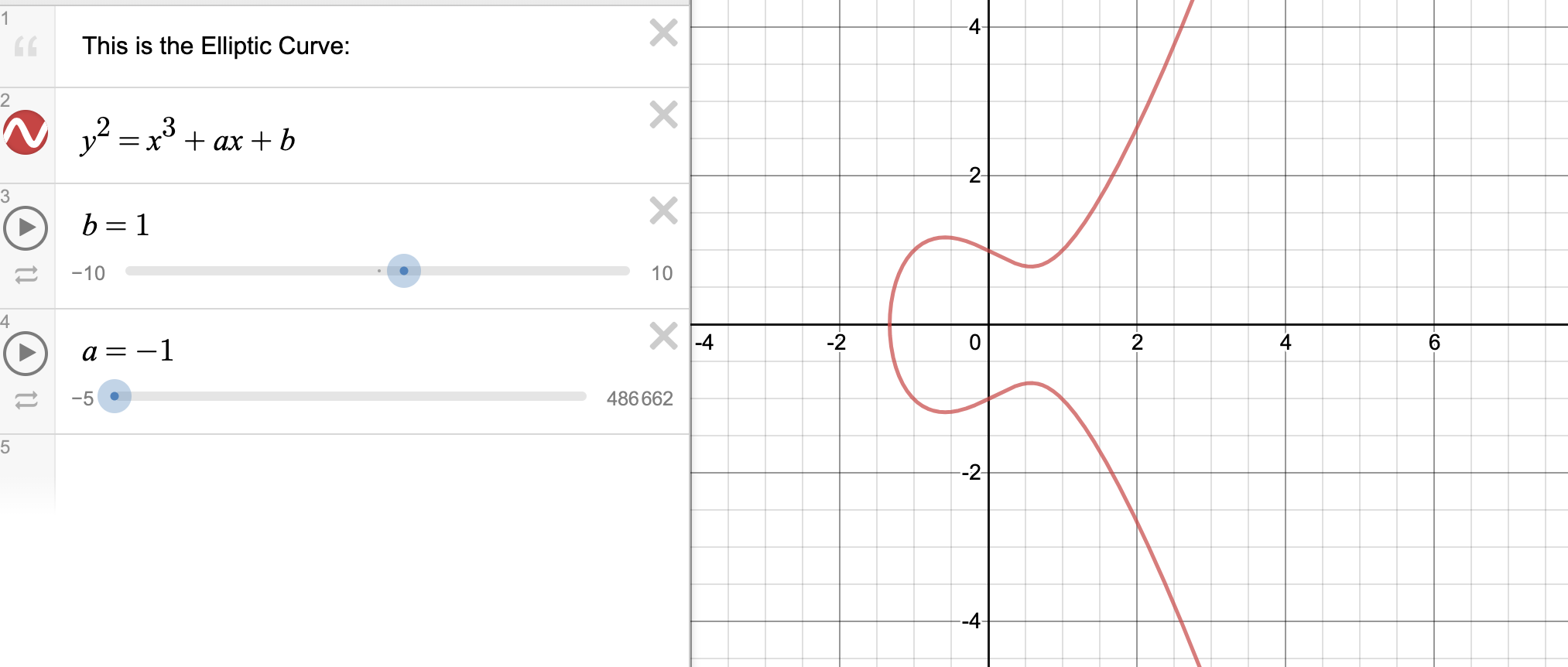$y^2 = x^3 + x + 1$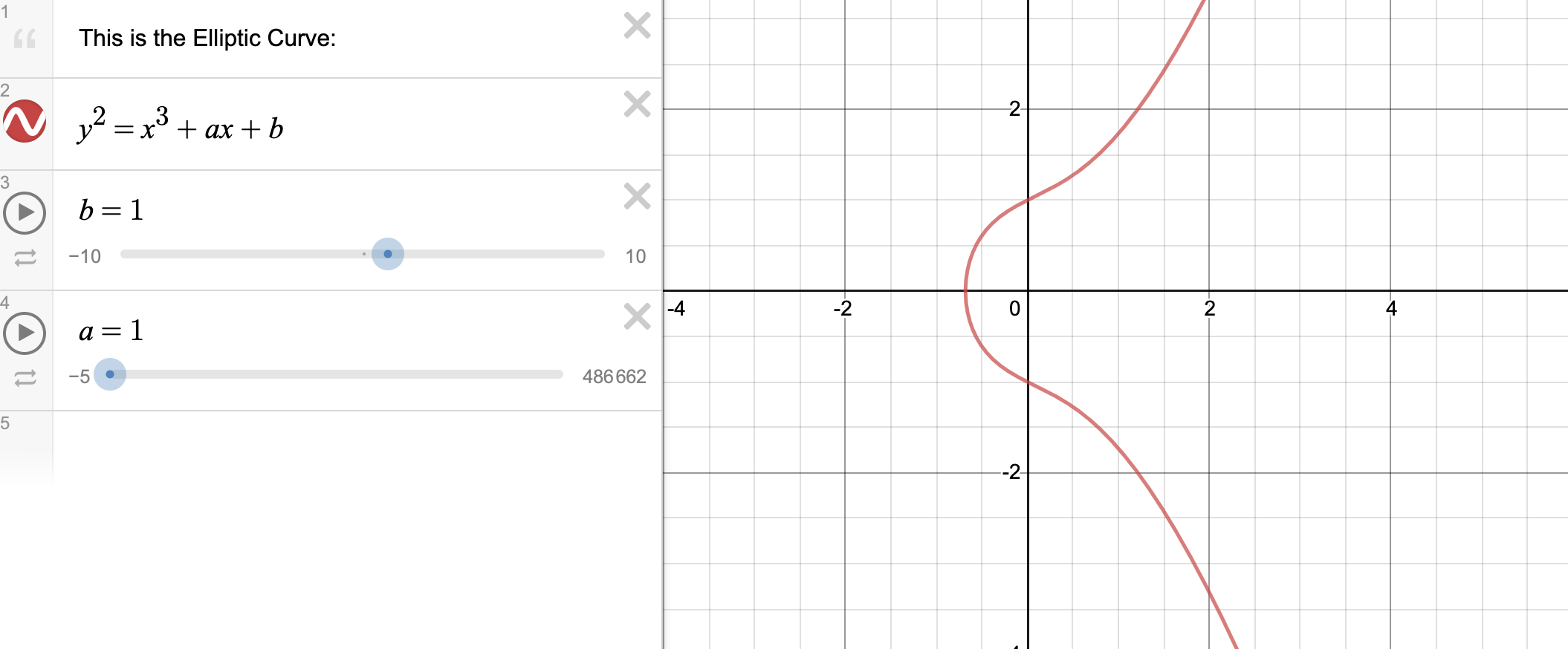#### 椭圆曲线运算 #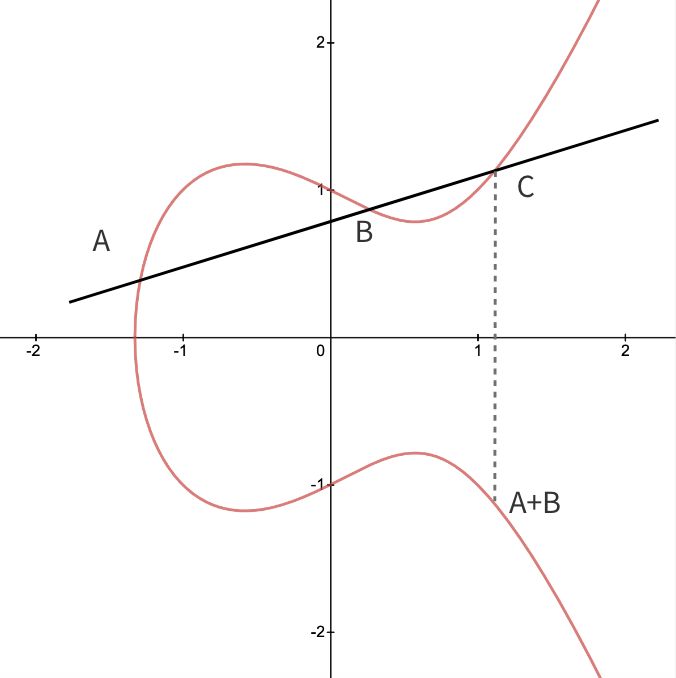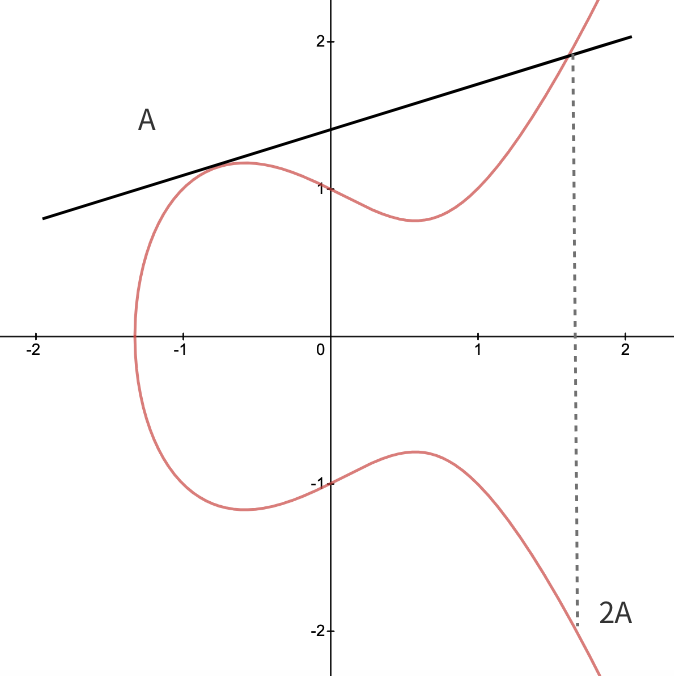A 关于 X 轴做对称得到的点定义为 -A。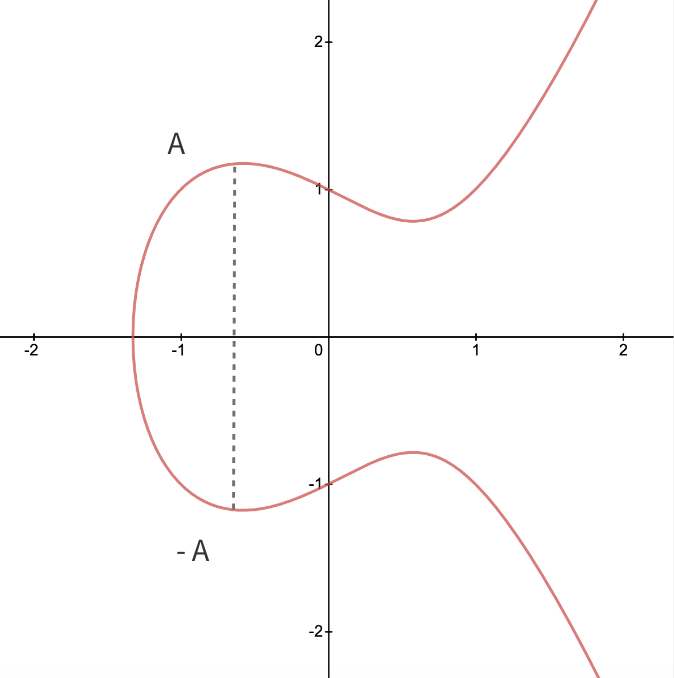A+B = C

A+C = D

A+D = E

A 和 B 重合演示如下：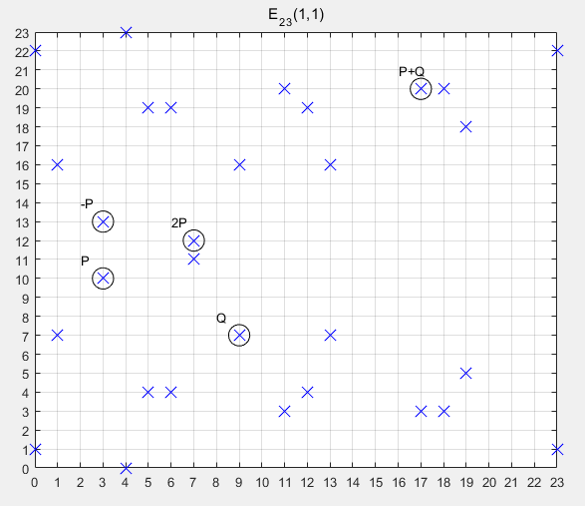1、李雷选择一条曲线 $Ep(a,b)$，取椭圆曲线上一点 $G$ 作为基点。

2、李雷选择一个私钥 $k$，然后生成公钥 $K=kG$

3、李雷把 $Ep(a,b)$ 、公钥 $K$ 和 基点 $G$ 传给韩梅梅

4、韩梅梅以上信息，把明文编码到 $Ep(a,b)$ 上一点 $M$，并产生一个随机数 $r$

5、韩梅梅计算 $C_1 = M+rK$ 和 $C_2 = rG$

6、韩梅梅把 $C_1、C_2$ 传给李雷

7、李雷收到信息后，计算 $C_1-kC2$ 结果就是点 $M$

#### 数学概念 #

##### 群 #

1、封闭性：如果 $a$ 和 $b$ 属于 $G$，那么 $a+b$ 也属于 $G$；

2、结合律：$(a+b)+c=a+(b+c)$；

3、存在单位元（注：在二元运算中，单位元指与任意元素运算不改变其值的元素，以实数为例，乘法单位元为1，加法单位元为0）$\theta$ 使得 $a+\theta = \theta + a = a$；

4、每个元素都存在逆元素，也即对于任意元素 $a$ 存在 $b$ 使得 $a+b=\theta$；

5、交换律：$a+b=b+a$

##### Curve25519 #

Curve25519 椭圆曲线方程为：$y^2 = x^3 + 486662x^2 + x$，使用基点 $x = 9$

## X.509 #

X.509 是密码学里公钥证书的格式标准。X.509 证书已应用在包括 TLS/SSL 在内的众多网络协议里，同时它也用在很多非在线应用场景里，比如电子签名服务。X.509 证书里含有公钥、身份信息（比如网络主机名，组织的名称或个体名称等）和签名信息（可以是证书签发机构 CA 的签名，也可以是自签名）。对于一份经由可信的证书签发机构签名或者可以通过其它方式验证的证书，证书的拥有者就可以用证书及相应的私钥来创建安全的通信，对文档进行数字签名。

## 参考文章 #

SSL证书CA机构

Curve25519

TLS的握手流程

ECC椭圆曲线加解密原理详解(配图)

ECC椭圆曲线加密算法：介绍

HTTPS 温故知新（五） —— TLS 中的密钥计算